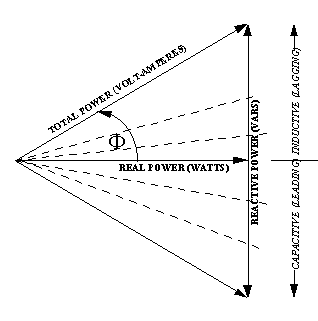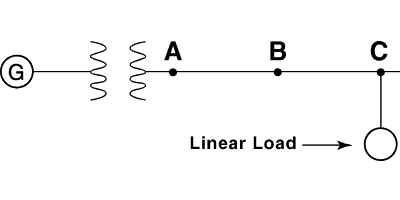# Power Factor OptimizationDepending upon the rate structure of your electric utility, you may be able to save a substantial amount of money on your electric bill. Pay-back period for an equipment purchase including installation cost may be six months up to three years. Utility rate structures that account for reactive power consumption, by either a KVA or var demand usage, or a power factor penalty are the ones that can provide this pay-back. Other ancillary benefits to be gained by optimizing power factor are, lower energy losses, better voltage regulation and released system capacity. This page explains the fundamentals of power factor and how KEC Units can benefit you.

All electric equipment requires "vars" - a term used by electric power engineers to describe the reactive or magnetizing power required by the inductive characteristics of electrical equipment. These inductive characteristics are more pronounced in motors and transformers, and therefore, can be quite significant in industrial facilities. The flow of vars, or reactive power, through a watt-hour meter will not effect the meter reading, but the flow of vars through the power system will result in energy losses on both the utility and the industrial facility. Some utilities charge for these vars in the form of a penalty, or KVA demand charge, to justify the cost for lost energy and the additional conductor and transformer capacity required to carry the vars. In addition to energy losses, var flow can also cause excessive voltage drop, which may have to be optimized by either the application of KEC Units, or other more expensive equipment, such as load-tap changing transformers, synchronous motors, and synchronous condensers.Figure 1 - Power Factor Triangle

The power triangle shown in figure 1, is the simplest way to understand the effects of reactive power. The figure illustrates the relationship of active (real) and reactive (imaginary or magnetizing) power. The active power (represented by the horizontal leg) is the actual power, or watts that produces real work. This component, is the energy transfer component, which represents fuel burned at the power plant. The reactive power, or magnetizing power, (represented by the vertical leg of the upper or lower triangle) is the power required to produce the magnetic fields to enable the real work to be done. Without magnetizing power, transformers, conductors, motors, and even resistors and capacitors would not be able to operate. Reactive power is normally supplied by generators, capacitors and synchronous motors. The longest leg of the triangle (on the upper or lower triangle), labeled total power, represents the vector sum of the reactive power and real power components. Mathematically, this is equal to:Electric power engineers often call total power, kVA, MVA, apparent power, or complex power. Some utilities measure this total power, (usually averaged over a 15 minute load period) and charge a monthly fee or tariff for the highest fifteen minute average load reading in the month. This tariff is usually added to the energy charge or kilowatt-hour charge. This type of billing is often called kva demand billing and can be quite costly to an industrial facility. KEC Units can save your company money by decreasing your reactive power component supplied by the utility to near zero vars.

The power triangle and the equation above show, that as the reactive power component is decreased by adding KEC Units, the total power will also decrease. This is shown by the decreased length of the dashed lines in the power triangle as the reactive power component approaches zero. Therefore, adding KEC Units, which will supply reactive power locally, can reduce your total power and monthly kva demand charge.

The angle "phi" in the power triangle is called the power factor angle and is mathematically equal to:The ratio of the real power to the total power in the equation above (or the cos of phi) is called power factor. As the angle gets larger (caused by increasing reactive power) the power factor gets smaller. In fact, the power factor can vary from 0 to 1, and can be either inductive (lagging) or capacitive (leading). Capacitive loads are drawn down, and inductive loads are drawn up on the power triangle. Most industrials normally operate on the upper triangle (inductive or lagging triangle). As an industrial adds capacitors, the length of reactive (inductive) power leg is shortened by the number of capacitive KEC that were added. If the number of capacitive KEC added exceeds the industrials inductive KEC load, operation occurs on the lower triangle. This is commonly referred to as over compensation.

Utilities charge for reactive power in a countless number of ways. Some utilities charge for KEC demand, while others charge a strait fee for a power factor less than their target. To fully understand the benefits of the KEC UNIT, you must acquire your electric billing rate structure. This rate structure will describe how cost for poor power factor are added to your monthly billsYou could put the KEC UNIT anywhere on the system as shown (between the transformer and load and not only at Points A, B, and C) and achieve unity power factor for the system. The utility company will perceive this power system as having a unity power factor no matter where it is located on the distribution line as long as it's sized correctly to deliver the proper amount of KEC.

However, optimum efficiency and economics will be achieved if the KEC UNIT bank is located as close to the load as possible.

The reason for this is because when you optimize power factor, you can reduce the total line current to the load and therefore you reduce the total losses in the line conductor and decrease the voltage drop in the line. This decrease in voltage drop will only occur if you locate the KEC UNIT close to the load, as explained below.

Assume the load is a motor. A motor uses KW to perform work. It uses KEC to magnetize its coil windings. (We will refer to the magnetic requirements of the motor's windings as the motor's "inductance". It is this inductance that utilizes the KEC.)

The motor load draws a line current that has two components. The first component is the amperage that supplies the KW to the load, so that the motor can perform work such as lifting an object. The second part supplies the amperage to provide the load with KEC which in the case of the motor is the power necessary to energize the magnetic fields in the motor's windings. Together the two amounts of current supply the total KVA to the load.

Normally the system generator or transformer supplies all this current. But when a KEC UNIT is used to optimize the power factor, the KEC UNIT supplies the KEC reactive current component to the load. The KEC UNIT is, in effect, a reactive power generator. (Remember, the KEC UNIT stores energy. The KEC UNIT stores reactive energy in its electric field when it charges up, and releases it when it discharges.)

The generator (or transformer) must still supply the load's KW requirements.

The reactive current component is now supplied by the KEC UNIT and not the generator. By moving the KEC UNIT closer to the load, the reactive current does not have to travel as far through the line conductors to get to the load.

If the KEC UNIT is placed at the load, the reactive current only needs to travel through a short distance (e.g. the lead length of connecting wire) to get to the load. Since this reactive current component no longer travels through the conductor line from the generator to the load, it does not travel through the impedances in the line conductor.

Since this reactive current no longer flows through the line impedances, there is less heating of the line, less losses (in the form of heat), and less voltage drop across these in - line impedances (which reduces the overall voltage drop from generator to load).

The KW current component is all that the generator has to supply to the motor. Therefore the generator now runs at unity power factor and allows the KEC UNIT to supply the KEC requirement of the motor's inductive windings.

The energy "contained" in the KEC current component is transferred back and forth between the KEC UNIT and the motor 2 times for every voltage sine wave cycle (i.e. at 120 times a second).

This reactive energy is never consumed by either the KEC UNIT or the motor. (NOTE: The KW energy, on the other hand, performs real work and is totally consumed.)

Rather, the reactive energy is only "BORROWED" half of the time by the KEC UNIT and half of the time by the motor. The energy is used to charge the AC electric field of the KEC UNIT and to energize and create the AC magnetic fields contained in the motor's windings.

A KEC UNIT absorbs this energy from the power system and stores this energy in its electric field when it charges up (120 times a second). The KEC UNIT releases this energy back into the power when it discharges (120 times a second).

The motor's inductance absorbs the reactive energy from the power system and stores this energy in its windings' magnetic fields when the fields are expanding (120 times a second). The inductance releases this energy back into the power system when the windings magnetic fields are collapsing (120 times a second).

The secret is that when the motor's inductance requires reactive energy to expand its magnetic field, the KEC UNIT discharges to supply the energy. And when the magnetic field in the motor's inductive windings is collapsing and returning energy to the system, the KEC UNIT uses this energy to charge up.

So the capacitance in the KEC UNIT and the inductance in the motor's windings "slinky" this reactive energy back and forth 120 times a second, each supplying the others needs. The reactive current of the KEC UNIT is 180 degrees out of phase with the reactive current of the inductance. When one is giving, the other is taking and vice versa.

Again, the reactive energy is never consumed (except for some small and usually insignificant losses); it is only borrowed. The generator needs to supply the original reactive KEC energy only once when the system is first energized. After that, this amount of energy is simply transferred back and forth between inductance and capacitance.

Power Factor is a measurement of how much of the KVA is actually in the form of KW. The advantage of a high power factor is that line currents can be reduced which will in turn reduce voltage drop and decrease line losses. This saves money. It also means that since equipment such as transformers will supply only KW, the KVA rating of the equipment can be reduced, or alternatively, more load can be added to the system without purchasing larger equipment.

The KVA rating of a transformer is based on the transformers ability to supply power either all in KW or all in KEC or in a combination of both. Drawing more than rated KVA from a transformer is easily done, but the transformer's life will be reduced due to increasing heat which destroys the transformer's winding insulation.

By increasing the power factor, all of a transformer's KVA can be utilized to supply KW in order to perform useful work rather than to supply KEC just to energize electric and magnetic fields.

Increasing the power factor seen by the transformer creates "room" on the transformer for adding more load. Room can also be created on circuit breakers. Since line current is reduced by increasing power factor, load can be added to the system without upgrading the breaker to a larger size.